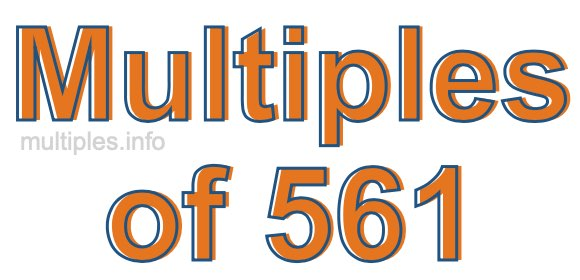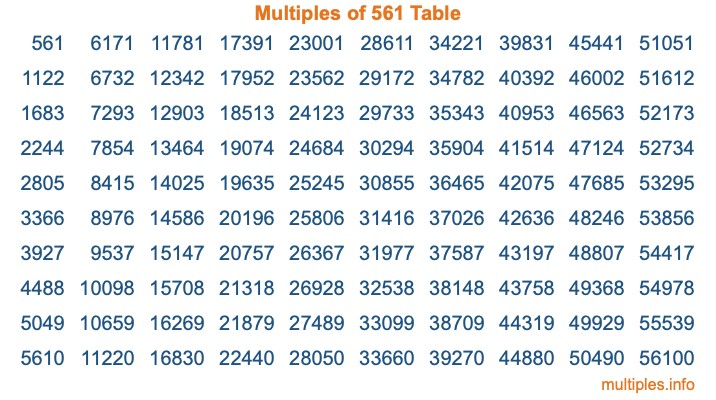Multiples of 561Welcome to the Multiples of 561 page. Here we will first teach you everything you will ever need to know about the multiples of 561, and then give you a study guide summary of everything we taught you to make sure you remember it all. Use this page to look up facts and learn information about the multiples of 561. This page will make you a multiples of five hundred sixty-one expert!

Definition of Multiples of 561
Multiples of 561 are all the numbers that when divided by 561 equal an integer. Each of the multiples of 561 are called a multiple. A multiple of 561 is created by multiplying 561 by an integer.

Therefore, to create a list of multiples of 561, you start with 1 multiplied by 561, then 2 multiplied by 561, then 3 multiplied by 561, and so on for as long as you want. Thus, the list of the first five multiples of 561 is 561, 1122, 1683, 2244, and 2805. To see a larger list of multiples of 561, see the printable image of Multiples of 561 further down on this page. We also have a category where you can choose any nth multiple of 561.

Multiples of 561 Checker
The Multiples of 561 Checker below checks to see if any number of your choice is a multiple of 561. In other words, it checks to see if there is any number (integer) that when multiplied by 561 will equal your number. To do that, we divide your number by 561. If the the quotient is an integer, then your number is a multiple of 561.

Is  a multiple of 561?

Least Common Multiple of 561 and ...
A Least Common Multiple (LCM) is the lowest multiple that two or more numbers have in common. This is also called the smallest common multiple or lowest common multiple and is useful to know when you are adding our subtracting fractions. Enter one or more numbers below (561 is already entered) to find the LCM.

Check out our LCM Calculator if you need more details about the Least Common Multiple or if you need the LCM for different numbers for adding and subtraction fractions.

nth Multiple of 561
As we stated above, 561 is the first multiple of 561, 1122 is the second multiple of 561, 1683 is the third multiple of 561, and so on. Enter a number below to find the nth multiple of 561.

th multiple of 561

Multiples of 561 vs Factors of 561
561 is a multiple of 561 and a factor of 561, but that is where the similarities end. All postive multiples of 561 are 561 or greater than 561. All positive factors of 561 are 561 or less than 561.

Below is the beginning list of multiples of 561 and the factors of 561 so you can compare:

Multiples of 561: 561, 1122, 1683, 2244, 2805, etc.

Factors of 561: 1, 3, 11, 17, 33, 51, 187, 561

As you can see, the multiples of 561 are all the numbers that you can divide by 561 to get a whole number. The factors of 561, on the other hand, are all the whole numbers that you can multiply by another whole number to get 561.

It's also interesting to note that if a number (x) is a factor of 561, then 561 will also be a multiple of that number (x).

Multiples of 561 vs Divisors of 561
The divisors of 561 are all the integers that 561 can be divided by evenly. Below is a list of the divisors of 561.

Divisors of 561: 1, 3, 11, 17, 33, 51, 187, 561

The interesting thing to note here is that if you take any multiple of 561 and divide it by a divisor of 561, you will see that the quotient is an integer.

Multiples of 561 Table
Below is an image of the first 100 multiples of 561 in a table. The table is in chronological order, column by column. The first column has the first ten multiples of 561, the second column has the next ten multiples of 561, and so on.The Multiples of 561 Table is also referred to as the 561 Times Table or Times Table of 561. You are welcome to print out our table for your studies.

Negative Multiples of 561
Although not often discussed or needed in math, it is worth mentioning that you can make a list of negative multiples of 561 by multiplying 561 by -1, then by -2, then by -3, and so on, to get the following list of negative multiples of 561:

-561, -1122, -1683, -2244, -2805, etc.

Multiples of 561 Summary
Below is a summary of important Multiples of 561 facts that we have discussed on this page. To retain the knowledge on this page, we recommend that you read through the summary and explain to yourself or a study partner why they hold true.

There are an infinite number of multiples of 561.

A multiple of 561 divided by 561 will equal a whole number.

561 divided by a factor of 561 equals a divisor of 561.

The nth multiple of 561 is n times 561.

The largest factor of 561 is equal to the first positive multiple of 561.

561 is a multiple of every factor of 561.

561 is a multiple of 561.

A multiple of 561 divided by a divisor of 561 equals an integer.

561 divided by a divisor of 561 equals a factor of 561.

Any integer times 561 will equal a multiple of 561.

Multiples of a Number
Here you can get the multiples of another number, all with the same attention to detail as we did for multiples of 561 on this page.

Multiples of
Multiples of 562
Did you find our page about multiples of five hundred sixty-one educational? Do you want more knowledge? Check out the multiples of the next number on our list!